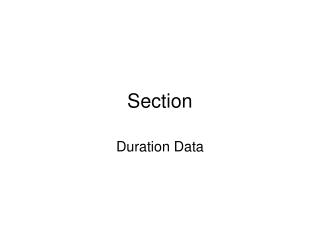# Section - PowerPoint PPT PresentationDownload PresentationSection

Section
Download Presentation## Section

- - - - - - - - - - - - - - - - - - - - - - - - - - - E N D - - - - - - - - - - - - - - - - - - - - - - - - - - -
##### Presentation Transcript

1. Section Duration Data

2. Introduction • Sometimes we have data on length of time of a particular event or ‘spells’ • Time until death • Time on unemployment • Time to complete a PhD • Techniques we will discuss were originally used to examine lifespan of objects like light bulbs or machines. These models are often referred to as “time to failure”

3. Notation • T is a random variable that indicates duration (time til death, find a new job, etc) • t is the realization of that variable • f(t) is a PDF that describes the process that determines the time to failure • CDF is F(t) represents the probability an event will happen by time t

4. F(t) represents the probability that the event happens by ‘t’. • What is the probability a person will die on or before the 65th birthday?

5. Survivor function, what is the chance you live past (t) • S(t) = 1 – F(t) • If 10% of a cohort dies by their 65th birthday, 90% will die sometime after their 65th birthday

6. Hazard function, h(t) • What is the probability the spell will end at time t, given that it has already lasted t • What is the chance you find a new job in month 12 given that you’ve been unemployed for 12 months already

7. PDF, CDF (Failure function), survivor function and hazard function are all related • λ(t) = f(t)/S(t) = f(t)/(1-F(t)) • We focus on the ‘hazard’ rate because its relationship to time indicates ‘duration dependence’

8. Example: suppose the longer someone is out of work, the lower the chance they will exit unemployment – ‘damaged goods’ • This is an example of duration dependence, the probability of exiting a state of the world is a function of the length

9. Mathematically • d λ(t) /dt = 0 then there is no duration dep. • d λ(t) /dt > 0 there is + duration dependence the probability the spell will end increases with time • d λ(t) /dt < 0 there is – duration dependence the probability the spell will end decreases over time

10. Your choice, is to pick values for f(t) that have +, - or no duration dependence

11. Different Functional Forms • Exponential • λ(t)= λ • Hazard is the same over time, a ‘memory less’ process • Weibull • F(t) = 1 – exp(-γtα) where α,γ > 0 • λ(t) = αγtα-1 • if α>1, increasing hazard • if α<1, decreasing hazard • if α=1, exponential

12. Others: Lognormal, log-logistic, Gompertz

13. NHIS Multiple Cause of Death • NHIS • annual survey of 60K households • Data on individuals • Self-reported healthm DR visits, lost workdays, etc. • MCOD • Linked NHIS respondents from 1986-1994 to National Death Index through Dec 31, 1995 • Identified whether respondent died and of what cause

14. Our sample • Males, 50-70, who were married at the time of the survey • 1987-1989 surveys • Give everyone 5 years (60 months) of followup

15. Key Variables • max_mths maximum months in the survey. • Diedin5 respondent died during the 5 years of followup • Note if diedn5=0, the max_mths=60. Diedin5 identifies whether the data is censored or not.

16. Identifying Duration Data in STATA • Need to identify which is the duration data stset length, failure(failvar) • Length=duration variable • Failvar=1 when durations end in failure, =0 for censored values • If all data is uncensored, omit failure(failvar)

17. In our case • Stset max_mths, failure(diedin5)

18. Getting Kaplan-Meier Curves • Tabular presentation of results sts list • Graphical presentation sts graph • Results by subgroup sts graph, by(income)